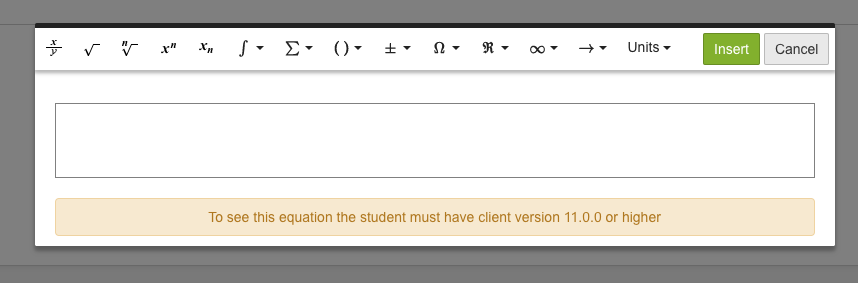Follow

# Use equation editor when creating questions

NOTE! To get the most out of the equation editor, the students need to have version 11.3.0 or later of the DigiExam client.

Earlier versions than 11.3.0 may experience problems with how the equations line up. Versions earlier than 11.0.0 will not be able to display equations at all from the equation editor.

The equation editor in DigiExam makes it possible to create questions with advanced equations. By clicking on the sum symbol in the text box where you type the question (see figure 1), a new box appears with the equation editor inside it.Figure 1

The equation editor that appears in the box (see Figure 2) supports the following among other things:

• Advanced mathematical functions such as integral sum and bionominal coefficient
• Set operators
• The most common mathematical operations

You can use the equation editor for all types of questions. In multiple choice questions and questions with multiple correct answers, you can only use characters from the equation editor on the question. You cannot use characters from the equation editor in the answer box.Figure 2